Courses

# RD Sharma Solutions -Ex-22.2, Data Handling Pictographs, Class 6, Maths Class 6 Notes | EduRev

## Class 6 : RD Sharma Solutions -Ex-22.2, Data Handling Pictographs, Class 6, Maths Class 6 Notes | EduRev

The document RD Sharma Solutions -Ex-22.2, Data Handling Pictographs, Class 6, Maths Class 6 Notes | EduRev is a part of the Class 6 Course RD Sharma Solutions for Class 6 Mathematics.
All you need of Class 6 at this link: Class 6

Q. 1. The following are the details or the number of students in a class of 30 students presents a week.

 Day Number of students present Monday 24 Tuesday 20 Wednesday 28 Thursday 30 Friday 26 Saturday 22

Represent the above data by a pictograph.

Sol:

Let an icon of a student represent 4 students. Then, the number of icons for each day is as follows:

 Day Number of students present Monday 24 / 4 = 6 Tuesday 20 / 4 = 5 Wednesday 28 / 4 = 7 Thursday 30 / 4 = 7.5 Friday 26 / 4 = 6.5 Saturday 22 / 4 = 5.5

The pictograph representing the above data is as follows: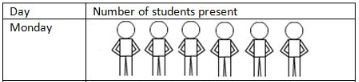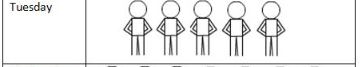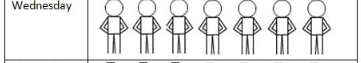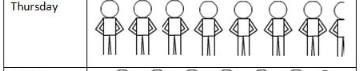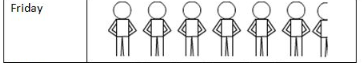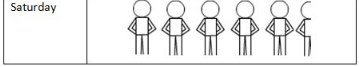Q. 2. Total number of students of a school in different years is shown in the following table:

 Year Number of students 1996 400 1998 550 2000 450 2002 600 2006 650

Represent the above data by a pictograph.

(a) Prepare a pictograph of students using one symbol an icon of a student to represent students and answer the following questions:

(i) How many symbols represent total number of students in the year 2002?

(ii) How many symbols represent total number of students for the year 1998?

(b) Prepare another pictograph of students using any other symbol each represents students. Which pictograph do you find more informative?

Sol:

(a) Let one icon represent 100 students.

Then, the numbers of icons for different years are as follows:

 Year Number of students 1996 400 / 100 = 4 1998 550 / 100 = 5.5 2000 450 / 100 = 4.5 2002 600 / 100 = 6 2006 650 / 100 = 6.5

The pictograph representing the above data is as follows: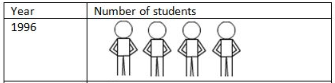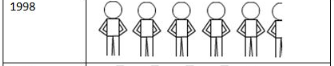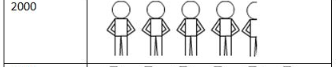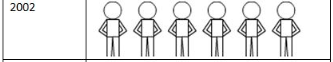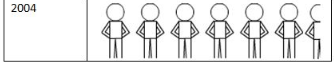(i) 6 icons represent the total number of students in the year 2002.

(ii) 5 and half icons represent the total number of students in the year 1998.

(b) Let one icon represent 50 students.

Then, the numbers of icons for different years are as follows:

 Year Number of students 1996 400 / 50 = 8 1998 550 / 50 = 11 2000 450 / 50 = 9 2002 600 / 50 = 12 2006 650 / 50 = 13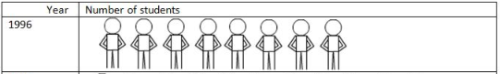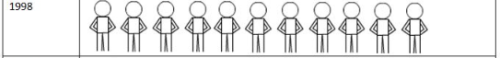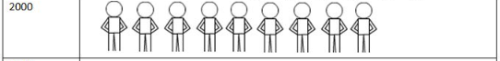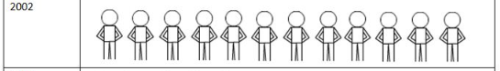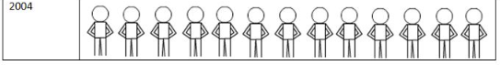Explanation: in the second pictograph, every icon is complete, therefore more convenient to understand.

Offer running on EduRev: Apply code STAYHOME200 to get INR 200 off on our premium plan EduRev Infinity!

103 docs

,

,

,

,

,

,

,

,

,

,

,

,

,

,

,

,

,

,

,

,

,

,

,

,

,

,

,

,

,

,

;